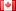# Revision history [back]

### Calculation taking too long, stats from scipy may be the problem?

Hey!

I have a question regarding a calculation I'm running. The code includes only 2 loops, one inside another, and some calculus inside. The loop run over many iterations (~100000X100000), but it is not an excuse for the calculation to be taking (so far...!) over 20 hours!

• The calcultation inside the loops is a fairly simple one: W[k]=W[k]+1/2...+..., but - i also include - sqrt(bla bla bla)stats.norm.rvs . One of the reasons for the ridiculously long calculation time, as I heard, might the calling for library from Scipy, where - as it means calling an outside library, which I don't know how it works, increeses the time of every step in the calculation in such a way that for such many iterations results in the long running time.

Can this be the source of the problem? Can calling an outside library, no matter if - Scipy of whatever else, increase the calculation time by so much?

What can I do?2 retaggedDSM5242 ●21 ●68 ●112

### Calculation taking too long, stats from scipy may be the problem?

Hey!

I have a question regarding a calculation I'm running. The code includes only 2 loops, one inside another, and some calculus inside. The loop run over many iterations (~100000X100000), but it is not an excuse for the calculation to be taking (so far...!) over 20 hours!

• The calcultation inside the loops is a fairly simple one: W[k]=W[k]+1/2...+..., but - i also include - sqrt(bla bla bla)stats.norm.rvs . One of the reasons for the ridiculously long calculation time, as I heard, might the calling for library from Scipy, where - as it means calling an outside library, which I don't know how it works, increeses the time of every step in the calculation in such a way that for such many iterations results in the long running time.

Can this be the source of the problem? Can calling an outside library, no matter if - Scipy of whatever else, increase the calculation time by so much?

What can I do?